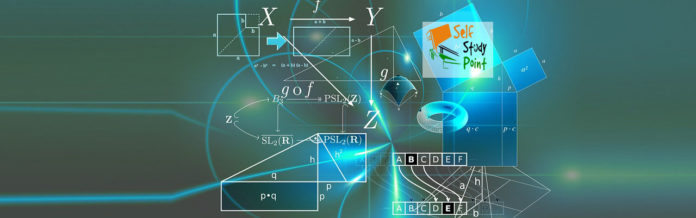Photoelectric effect thus gave evidence to the strange fact that light in interaction with matter behaved as if it was made of quanta or packets of energy, each of energy h ν.

We can summaries the photon picture of electromagnetic radiation as follows:

1. In the interaction of radiation with matter, radiation behaves as if it is made up of particles called photons.
2. Each photon has energy E (=hν) and momentum p (= h ν/c), and speed c, the speed of light.
3. All photons of light of a particular frequency ν, or wavelength λ, have the same energy E (=hν = hc/λ) and momentum p (= hν/c = h/λ), whatever the intensity of radiation may be. By increasing the intensity of light of given wavelength, there is only an increase in the number of photons per second crossing a given area, with each photon having the same energy. Thus, photon energy is independent of intensity of radiation.
4. Photons are electrically neutral and are not deflected by electric and magnetic fields.
5. In a photon-particle collision (such as photon-electron collision), the total energy and total momentum are conserved. However, the number of photons may not be conserved in a collision. The photon may be absorbed or a new photon may be created.